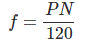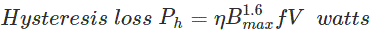# Losses in DC Machine

As we know “Energy neither can be created nor it can be destroyed, it can only be transferred from one form to another”. In DC machine, mechanical energy is converted into the electrical energy. During this process, the total input power is not transformed into output power. Some part of input power gets wasted in various forms. The form of this loss may vary from one machine to another. These losses give in the rise in temperature of the machine and reduce the efficiency of the machine. In DC Machine, there are broadly four main categories of energy loss.

## Copper Losses or Electrical Losses in DC Machine or Winding Loss

The copper losses are the winding losses taking place during the current flowing through the winding. These losses occur due to the resistance in the winding. In a DC machine, there are only two windings, armature and field winding.
Thus copper losses categories in three parts; armature loss, the field winding loss, and brush contact resistance loss. The copper losses are proportional to the square of the current flowing through the winding.

### Armature Copper Loss in DC Machine

Armature copper loss = Ia2Ra
Where, Ia is armature current and Ra is armature resistance.

### Field Winding Copper Loss in DC Machine

Field winding copper loss = If2Rf
Where, If is field current and Rf is field resistance.
These losses are about 25% theoretically, but practically it is constant.

### Brush Contact Resistance Loss in DC Machine

Brush contact loss attributes to resistance between the surface of brush and commutator. It is not a loss which could be calculated separately as it is a part of variable losses. Generally, it contributes to both the types of copper losses. So, they are factors in the calculation of the above losses.

## Core Losses or Iron Losses in DC Machine or Magnetic Losses

As iron core of the armature is rotating in magnetic field, some losses occurs in the core which is called core losses. Normally, machines are operated with constant speed, so these losses are almost constant. These losses are categorized in two form; Hysteresis loss and Eddy current loss.

### Hysteresis Loss in DC Machine

Hysteresis losses occur in the armature winding due to reversal of magnetization of the core. When the core of the armature exposed to the magnetic field, it undergoes one complete rotation of magnetic reversal. The portion of the armature which is under S-pole, after completing half electrical revolution, the same piece will be under the N-pole, and the magnetic lines are reversed in order to overturn the magnetism within the core. The constant process of magnetic reversal in the armature, consume some amount of energy which is called hysteresis loss. The percentage of loss depends upon the quality and volume of the iron.

#### The Frequency of Magnetic ReversalWhere,
P = Number of poles
N = Speed in rpm

#### Steinmetz Formula

The Steinmetz formula is for the calculation of hysteresis loss.Where,
η = Steinmetz hysteresis co-efficient
Bmax = Maximum flux Density in armature winding
F = Frequency of magnetic reversals
V = Volume of armature in m3.

### Eddy Current Loss in DC Machine

According to Faraday’s law of electromagnetic induction, when an iron core rotates in the magnetic field, an emf is also induced in the core. Similarly, when armature rotates in the magnetic field, the small amount of emf induced in the core which allows the flow of charge in the body due to the conductivity of the core. This current is useless for the machine. This loss of current is called eddy current. This loss is almost constant for the DC machines. It could be minimized by selecting the laminated core.

## Mechanical Losses in DC Machine

The losses associated with mechanical friction of the machine are called mechanical losses. These losses occur due to friction in the moving parts of the machine like bearing, brushes etc, and windage losses occur due to the air inside the rotating coil of the machine. These losses are usually very small about 15% of full load loss.

## Stray Load Losses in DC Machine

There are some more losses other than the losses which have been discussed above. These losses are called stray-load losses. These miscellaneous losses are due to the short-circuit current in the coil undergoing commutation, distortion of flux due to the armature and many more losses which are difficult to find. These losses are difficult to determine. However, they are taken as 1% of the whole load power output.

Want To Learn Faster? 🎓
Get electrical articles delivered to your inbox every week.
No credit card required—it’s 100% free.